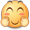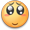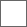### 拼图攻略先开一贴 楼主去吃饭 人多回来就更gkd，拼图搞快点3 5 1

0 7 2
4 6 8#include<stdio.h>
#include<stdlib.h>
int i,j,a,x,y,step=0,dx={-1,0,1,0},dy={0,-1,0,1},min=50,b,c;
int check()
{
int i,j;
for(i=1;i<=3;i++)for(j=1;j<=3;j++)if(i==3&&j==3)return 1;else if(a[i][j]!=3*i+j-3)return 0;
}
void dfs(int x,int y)
{
int ii,xx,yy;
if(step>=min)return;
if(check()==1){min=step;for(ii=0;ii<=step;ii++)c[ii]=b[ii];return;}
for(ii=0;ii<4;ii++)
{
xx=x+dx[ii];yy=y+dy[ii];
if(xx>=1&&xx<=3&&yy<=3&&yy>=1&&((step==0)||(3*xx+yy-3!=b[step-1])))
{
a[x][y]=a[xx][yy];
a[xx][yy]=0;
step++;b[step]=3*xx+yy-3;
dfs(xx,yy);
step--;
a[xx][yy]=a[x][y];
a[x][y]=0;
}
}
}
int main(){
for(i=1;i<=3;i++)for(j=1;j<=3;j++)scanf("%d",&a[i][j]);
for(i=1;i<=3;i++)for(j=1;j<=3;j++)if(a[i][j]==0){x=i;y=j;}
b=3*x+y-3;dfs(x,y);
printf("%d\n",min);
for(i=0;i<=min;i++)printf("%d ",c[i]);
return 0;
}#### 扫二维码下载贴吧客户端